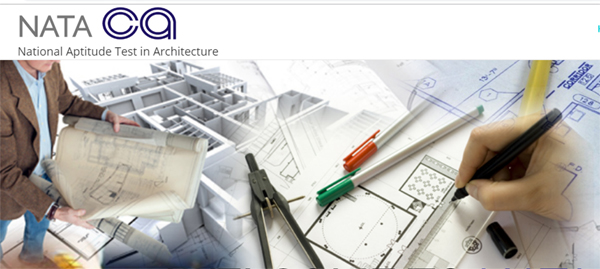# आर्किटेक्चर में बनाना है कैरियर तो NATA के लिए करें आवेदन

National Aptitude Test in Architecture(NATA)  के लिए 21 जनवरी से शुरू होंगे आवेदनअगर आप 12वीं के बाद आर्किटेक्चर के क्षेत्र में कैरियर बनाना चाहते हैं तो नेशनल एप्टीट्यूड टेस्ट इन आर्किटेक्चर (नाटा) आपके लिए बेहतर विकल्प हो सकता है। काउंसिल ऑफ आर्किटेक्चर (COA) की ओर से यह परीक्षा कराई जाती है।

12वीं के बाद पांच वर्षीय आर्किटेक्चर कोर्स के लिए इस परीक्षा से एडमिशन की राह खुलती है। इस बार भी इसके दो टेस्ट लिए जाएंगे। पहला टेस्ट 14 अप्रैल 2019 को होगा। दूसरा टेस्ट 07 जुलाई 2019 को होगा।

पहले टेस्ट के लिए 24 जनवरी से 11 मार्च तक आवेदन करने का मौका मिलेगा। दूसरे टेस्ट के लिए 24 जनवरी से 12 जून तक आवेदन करने का मौका मिलेगा। इस परीक्षा से देशभर के संस्थानों में बीआर्क करने का मौका मिलेगा।

यह योग्यता है जरूरी

आवेदक का किसी मान्यता प्राप्त बोर्ड से 12वीं पास होना जरूरी है। या 10वीं के बाद तीन वर्षीय डिप्लोमा पास होना जरूरी है। 12वीं में मैथ्स के साथ कम से कम 50 परसेंट मार्क्स होने जरूरी हैं।

यह होगा टेस्ट का सिलेबस

MATHEMATICS

Algebra: Definitions of A. P. and G.P.; General term; Summation of first n-terms of series ∑n, ∑n²,∑n3 ;

Arithmetic/Geometric series, A.M., G.M. and their relation; Infinite G.P. series and its sum.

Logarithms: Definition; General properties; Change of base.

Matrices: Concepts of m x n (m ≤ 3, n ≤ 3) real matrices, operations of addition, scalar multiplication and

multiplication of matrices. Transpose of a matrix. Determinant of a square matrix. Properties of determinants

(statement only). Minor, cofactor and adjoint of a matrix. Nonsingular matrix. Inverse of a matrix. Finding area

of a triangle. Solutions of system of linear equations. (Not more than 3 variables).

Trigonometry: Trigonometric functions, addition and subtraction formulae, formulae involving multiple and

submultiple angles, general solution of trigonometric equations. Properties of triangles, inverse trigonometric

functions and their properties.

Coordinate geometry: Distance formula, section formula, area of a triangle, condition of collinearity of three

points in a plane. Polar coordinates, transformation from Cartesian to polar coordinates and vice versa. Parallel

transformation of axes, concept of locus, elementary locus problems. Slope of a line. Equation of lines in

different forms, angle between two lines. Condition of perpendicularity and parallelism of two lines. Distance

of a point from a line. Distance between two parallel lines. Lines through the point of intersection of two lines.

Equation of a circle with a given center and radius. Condition that a general equation of second degree in x, y

may represent a circle. Equation of a circle in terms of endpoints of a diameter . Equation of tangent, normal

and chord. Parametric equation of a circle. Intersection of a line with a circle. Equation of common chord of

two intersecting circles.

3-Dimensional Co-ordinate geometry: Direction cosines and direction ratios, distance between two points

and section formula, equation of a straight line, equation of a plane, distance of a point from a plane.

Theory of Calculus: Functions, composition of two functions and inverse of a function, limit, continuity,

derivative, chain rule, derivative of implicit functions and functions defined parametrically. Integration as a

reverse process of differentiation, indefinite integral of standard functions. Integration by parts. Integration

by substitution and partial fraction. Definite integral as a limit of a sum with equal subdivisions. Fundamental

theorem of integral calculus and its applications. Properties of definite integrals. Formation of ordinary

differential equations, solution of homogeneous differential equations, separation of variables method, linear

first order differential equations.

Application of Calculus: Tangents and normals, conditions of tangency. Determination of monotonicity,

maxima and minima. Differential coefficient as a measure of rate. Motion in a straight line with constant

acceleration. Geometric interpretation of definite integral as area, calculation of area bounded by elementary

curves and Straight lines. Area of the region included between two elementary curves.

Permutation and combination: Permutation of n different things taken r at a time (r ≤ n). Permutation of n

things not all different. Permutation with repetitions (circular permutation excluded). Combinations of n

different things taken r at a time (r ≤ n). Combination of n things not all different. Basic properties. Problems

involving both permutations and combinations.

Statistics and Probability: Measure of dispersion, mean, variance and standard deviation, frequency

distribution. Addition and multiplication rules of probability, conditional probability and Bayes’ Theorem,

independence of events, repeated independent trails and Binomial distribution.

GENERAL APTITUDE

Objects, texture related to architecture and built environment. Interpretation of pictorial compositions,

Visualizing three-dimensional objects from two-dimensional drawing. Visualizing different sides of 3D objects.

Analytical reasoning, mental ability (visual, numerical and verbal), General awareness of national/

international architects and famous architectural creations.

Mathematical reasoning: Statements, logical operations like and, or, if and only if, implies, implied by.

Understanding of tautology, converse, contradiction and contrapositive.

Sets and Relations: Idea of sets, subsets, power set, complement, union, intersection and difference of sets,

Venn diagram, De Morgan’s Laws, Relation and its properties. Equivalence relation — definition and

elementary examples.

DRAWING TEST

Understanding of scale and proportion of objects, geometric composition, shape, building forms and

elements, aesthetics, colour texture, harmony and contrast. Conceptualization and Visualization through

structuring objects in memory. Drawing of patterns – both geometrical and abstract. Form transformations in

2D and 3D like union, subtraction, rotation, surfaces and volumes. Generating plan, elevation and 3D views of

objects. Creating 2D and 3D compositions using given shape and forms. Perspective drawing, Sketching of

urbanscape and landscape, Common day-to-day life objects like furniture, equipment etc., from memory.

NATA 2019 के आवेदन के लिए क्लिक करें

12वीं के बाद एडमिशन की और जानकारी को क्लिक करें

Posted Under
Tagged